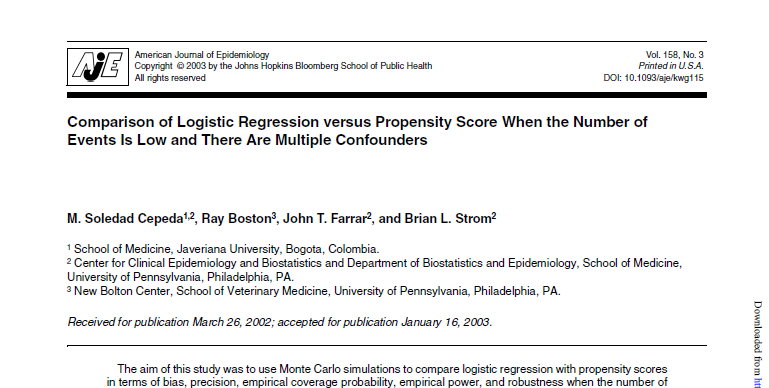# Recommended: Comparison of Logistic Regression versus Propensity Score When the Number of Events Is Low and There Are Multiple Confounders

Propensity scores represent an intriguing alternative method to reduce the impact of confounding variables, especially when there are multiple potential confounding variables. This paper considers a range of models comparing the propensity score approach to the more traditional approaches of adjusting for confounders. I think the conclusions are overly simplistic, but the paper is still worth reading.

Cepeda MS. Comparison of Logistic Regression versus Propensity Score When the Number of Events Is Low and There Are Multiple Confounders. American Journal of Epidemiology. 2003;158(3):280–287. doi:10.1093/aje/kwg115. Abstract: “The aim of this study was to use Monte Carlo simulations to compare logistic regression with propensity scores in terms of bias, precision, empirical coverage probability, empirical power, and robustness when the number of events is low relative to the number of confounders. The authors simulated a cohort study and performed 252,480 trials. In the logistic regression, the bias decreased as the number of events per confounder increased. In the propensity score, the bias decreased as the strength of the association of the exposure with the outcome increased. Propensity scores produced estimates that were less biased, more robust, and more precise than the logistic regression estimates when there were seven or fewer events per confounder. The logistic regression empirical coverage probability increased as the number of events per confounder increased. The propensity score empirical coverage probability decreased after eight or more events per confounder. Overall, the propensity score exhibited more empirical power than logistic regression. Propensity scores are a good alternative to control for imbalances when there are seven or fewer events per confounder; however, empirical power could range from 35% to 60%. Logistic regression is the technique of choice when there are at least eight events per confounder.” Available at: http://europepmc.org/abstract/MED/12882951